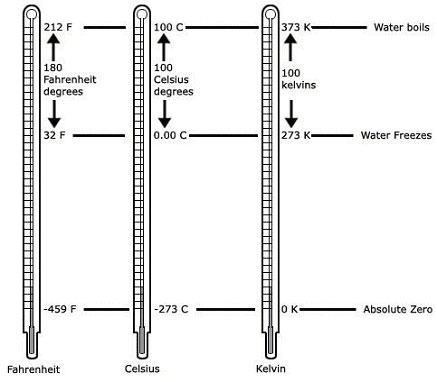A# units of heatComparison of the Fahrenheit, Celsius, and Kelvin scales. Credit: NASA .

## Celsius

The Celsius, also known as centigrade, is a unit of temperature difference equal to 1 kelvin (K), originally defined by dividing the interval between the boiling point and freezing point of water into 100 equal divisions. The name 'Celsius' officially replaced 'centigrade' in 1948. The Celsius scale has largely superceded the Fahrenheit scale.

## Fahrenheit

The Fahrenheit scale is a temperature scale based on the freezing point of water (32°F) and the boiling point of water (212°F). The interval between these points is divided into 180 equal parts. Although still commonly used in the United States, elsewhere the Fahrenheit scale has been superceded by the Celsius first proposed by Anders Celsius. Degrees Fahrenheit are converted to degrees Celsius by subtracting 32 and then dividing by 1.8. See also third law of thermodynamics.

## kelvin

The kelvin (K) is the base unit of thermodynamic temperature in the SI system of units. It is equal to the fraction 1/273.16 of the thermodynamic temperature of the triple point of water. One kelvin corresponds to an interval of one degree on the Celsius (centigrade) scale, so that the freezing and boiling points of water, at standard pressure, are 273 K and 373 K, respectively. The temperature of absolute zero is 0 K (-273.16°C). The unit is named after the Scottish physicist Lord Kelvin (William Thomson).